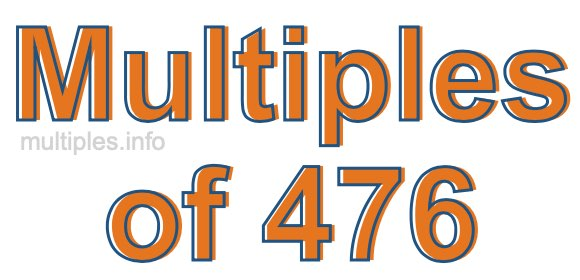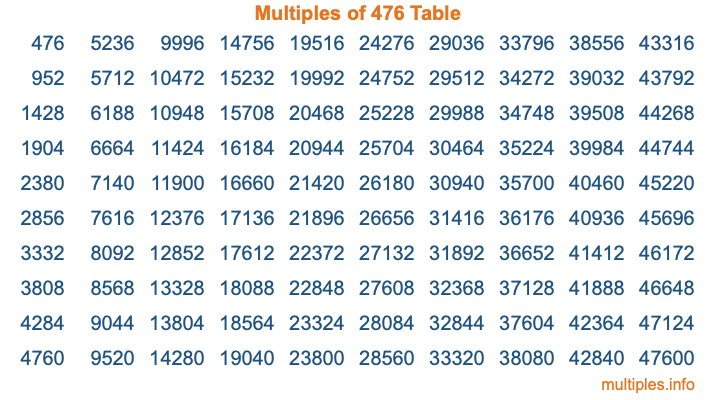Multiples of 476Welcome to the Multiples of 476 page. Here we will first teach you everything you will ever need to know about the multiples of 476, and then give you a study guide summary of everything we taught you to make sure you remember it all. Use this page to look up facts and learn information about the multiples of 476. This page will make you a multiples of four hundred seventy-six expert!

Definition of Multiples of 476
Multiples of 476 are all the numbers that when divided by 476 equal an integer. Each of the multiples of 476 are called a multiple. A multiple of 476 is created by multiplying 476 by an integer.

Therefore, to create a list of multiples of 476, you start with 1 multiplied by 476, then 2 multiplied by 476, then 3 multiplied by 476, and so on for as long as you want. Thus, the list of the first five multiples of 476 is 476, 952, 1428, 1904, and 2380. To see a larger list of multiples of 476, see the printable image of Multiples of 476 further down on this page. We also have a category where you can choose any nth multiple of 476.

Multiples of 476 Checker
The Multiples of 476 Checker below checks to see if any number of your choice is a multiple of 476. In other words, it checks to see if there is any number (integer) that when multiplied by 476 will equal your number. To do that, we divide your number by 476. If the the quotient is an integer, then your number is a multiple of 476.

Is  a multiple of 476?

Least Common Multiple of 476 and ...
A Least Common Multiple (LCM) is the lowest multiple that two or more numbers have in common. This is also called the smallest common multiple or lowest common multiple and is useful to know when you are adding our subtracting fractions. Enter one or more numbers below (476 is already entered) to find the LCM.

Check out our LCM Calculator if you need more details about the Least Common Multiple or if you need the LCM for different numbers for adding and subtraction fractions.

nth Multiple of 476
As we stated above, 476 is the first multiple of 476, 952 is the second multiple of 476, 1428 is the third multiple of 476, and so on. Enter a number below to find the nth multiple of 476.

th multiple of 476

Multiples of 476 vs Factors of 476
476 is a multiple of 476 and a factor of 476, but that is where the similarities end. All postive multiples of 476 are 476 or greater than 476. All positive factors of 476 are 476 or less than 476.

Below is the beginning list of multiples of 476 and the factors of 476 so you can compare:

Multiples of 476: 476, 952, 1428, 1904, 2380, etc.

Factors of 476: 1, 2, 4, 7, 14, 17, 28, 34, 68, 119, 238, 476

As you can see, the multiples of 476 are all the numbers that you can divide by 476 to get a whole number. The factors of 476, on the other hand, are all the whole numbers that you can multiply by another whole number to get 476.

It's also interesting to note that if a number (x) is a factor of 476, then 476 will also be a multiple of that number (x).

Multiples of 476 vs Divisors of 476
The divisors of 476 are all the integers that 476 can be divided by evenly. Below is a list of the divisors of 476.

Divisors of 476: 1, 2, 4, 7, 14, 17, 28, 34, 68, 119, 238, 476

The interesting thing to note here is that if you take any multiple of 476 and divide it by a divisor of 476, you will see that the quotient is an integer.

Multiples of 476 Table
Below is an image of the first 100 multiples of 476 in a table. The table is in chronological order, column by column. The first column has the first ten multiples of 476, the second column has the next ten multiples of 476, and so on.The Multiples of 476 Table is also referred to as the 476 Times Table or Times Table of 476. You are welcome to print out our table for your studies.

Negative Multiples of 476
Although not often discussed or needed in math, it is worth mentioning that you can make a list of negative multiples of 476 by multiplying 476 by -1, then by -2, then by -3, and so on, to get the following list of negative multiples of 476:

-476, -952, -1428, -1904, -2380, etc.

Multiples of 476 Summary
Below is a summary of important Multiples of 476 facts that we have discussed on this page. To retain the knowledge on this page, we recommend that you read through the summary and explain to yourself or a study partner why they hold true.

There are an infinite number of multiples of 476.

A multiple of 476 divided by 476 will equal a whole number.

476 divided by a factor of 476 equals a divisor of 476.

The nth multiple of 476 is n times 476.

The largest factor of 476 is equal to the first positive multiple of 476.

476 is a multiple of every factor of 476.

476 is a multiple of 476.

A multiple of 476 divided by a divisor of 476 equals an integer.

476 divided by a divisor of 476 equals a factor of 476.

Any integer times 476 will equal a multiple of 476.

Multiples of a Number
Here you can get the multiples of another number, all with the same attention to detail as we did for multiples of 476 on this page.

Multiples of
Multiples of 477
Did you find our page about multiples of four hundred seventy-six educational? Do you want more knowledge? Check out the multiples of the next number on our list!